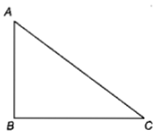NEET Questions Solved

NEET - 2011

A current carrying closed loop in the form of a right angle isosceles triangle ABC is placed in a uniform magnetic field acting along AB. If the magnetic force on the arm BC is F, the force on the arm AC is(a) $\mathbf{-}\mathbf{F}$                                               (b) $\mathbf{F}$

(c) $\sqrt{2}\mathbf{F}$                                             (d) $-\sqrt{2}\mathbf{F}$${\mathbf{F}}_{\mathrm{AB}}=0$

Difficulty Level:

• 33%
• 17%
• 34%
• 18%